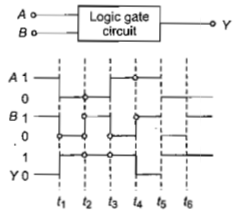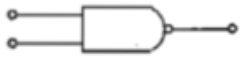Which one of the following statement is false?

(a) Pure Si doped with trivalent impurities gives a p-type semiconductor

(b) Majority carries in a n-type semiconductor are holes

(c) Minority carries in a p-type semiconductor are electrons

(d) The resistance of intrinsic semiconductor decreases with increase of temperature

Concept Questions :-

Types of semiconductors
High Yielding Test Series + Question Bank - NEET 2020

Difficulty Level:

The device that can act as a complete electronic circuit is

1. Junction diode

2. Integrated circuit

3. Junction transistor

4. Zener diode

Concept Questions :-

Transistor
High Yielding Test Series + Question Bank - NEET 2020

Difficulty Level:

A common emitter amplifier has a voltage gain of 50, an input impedance of 100$\mathrm{\Omega }$ and an output impedance of 200$\mathrm{\Omega }$. The power gain the amplifier is

(a) 500         (b) 1000

(c) 1250       (d) 50

Concept Questions :-

Applications of Transistor
High Yielding Test Series + Question Bank - NEET 2020

Difficulty Level:

The following figure shows a logic gate circuit with two inputs A and B and the output Y. The voltage waveforms of A, B and Y are as givenThe logic gate is

(a) $\mathrm{NOR}$ gate                                                 (b) $\mathrm{OR}$ gate

(c)  gate                                                 (d) gate

Concept Questions :-

Logic gates
High Yielding Test Series + Question Bank - NEET 2020

Difficulty Level:

For transistor action

(1) Base, emitter and collector regions should have similar size and doping concentrations.

(2) The base regions must be very thin and lightly doped.

(3) The emitter-base junction is forward biased and the base-collector junction is reverse biased.

(4) Both the emitter-base junction as well as the base-collector junction are forward biased.

Which of the following pairs of statements is correct?

(a)   (4) and (1)                                       (b)   (1) and (2)

(c)   (2) and (3)                                       (d)    (3) and (4)

Concept Questions :-

Transistor
High Yielding Test Series + Question Bank - NEET 2020

Difficulty Level:

A p-n photodiode is fabricated from a semiconductor with a band gap of  It can detect a signal of wavelength

(a)                                     (b)

(c)                                   (d)

Concept Questions :-

Applications of PN junction
High Yielding Test Series + Question Bank - NEET 2020

Difficulty Level:

The symbolic representation of four logic gates(i)                                                                              (ii)(iii)                                                                              (iv)

The logic symbols for $\mathrm{OR},$ $\mathrm{NOT}$ and $\mathrm{NAND}$ gates are respectively

(a)    (iii), (iv), (ii)                                            (b)   (iv), (i), (iii)

(c)    (iv), (ii), (i)                                              (d)   (i), (iii), (iv)

Concept Questions :-

Logic gates

Difficulty Level:

A transistor is operated in common-emitter configuration at ${V}_{c}=2volt$ such that a change in the base current from 100 $\mu A$ to 200 $\mu A$ produces a change in the collector current from 5 mA to 10 mA. The current gain is

1. 75                                2. 100

3. 150                              4. 50

Concept Questions :-

Transistor
High Yielding Test Series + Question Bank - NEET 2020

Difficulty Level:

Sodium has body centred packing.Distance between two nearest atom is $3.7\stackrel{0}{A}$. The lattice parameter is

(a) 6.8 $\stackrel{0}{A}$                                     (b) 4.3 $\stackrel{0}{A}$

(c) 3.0 $\stackrel{0}{A}$                                     (d) 8.6 $\stackrel{0}{A}$

Concept Questions :-

Types of semiconductors
High Yielding Test Series + Question Bank - NEET 2020

Difficulty Level:

If the lattice parameter for a crystalline structure is  then the atomic radius in fcc crystal is

(a)                                   (b)

(c)                                 (d)

Concept Questions :-

Types of semiconductors